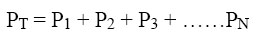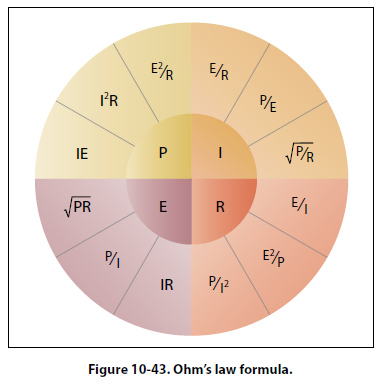Power in a Series and Parallel Circuit The total power dissipated in both a series and parallel circuit is equal to the sum of the power dissipated in each resistor in the circuit. Power is simply additive and can be stated as:Figure 10-43 provides a summary of all the possible transpositions of the Ohm’s law formula and the power formula.Energy in an Electrical Circuit Energy is defined as the ability to do work. Because power is the rate of energy usage, power used over a span of time is actually energy consumption. If power and time are multiplied together, we will get energy. The joule is defined as a unit of energy. There is another unit of measure which is perhaps more familiar. Because power is expressed in watts and time in seconds, a unit of energy can be called a watt-second (Ws) or more recognizable from the electric bill, a kilowatt-hour (kWh). Refer to Page 3-3 for further discussion on energy. Sources of Electricity Electrical energy can be produced in a number of methods. The four most common are pressure, chemical, thermal, and light. Pressure Source This form of electrical generation is commonly known as piezoelectric (piezo or piez taken from Greek: to press; pressure; to squeeze) is a result of the application of mechanical pressure on a dielectric or nonconducting crystal. The most common piezoelectric materials used today are crystalline quartz and Rochelle salt. However, Rochelle salt is being superseded by other materials, such as barium titanate. The application of a mechanical stress produces an electric polarization, which is proportional to this stress. This polarization establishes a voltage across the crystal. If a circuit is connected across the crystal a flow of current can be observed when the crystal is loaded (pressure is applied). An opposite condition can occur, where an application of a voltage between certain faces of the crystal can produce a mechanical distortion. This effect is commonly referred to as the piezoelectric effect. Piezoelectric materials are used extensively in transducers for converting a mechanical strain into an electrical signal. Such devices include microphones, phonograph pickups and vibration-sensing elements. The opposite effect, in which a mechanical output is derived from an electrical signal input, is also widely used in headphones and loudspeakers. Chemical Source Chemical energy can be converted into electricity; the most common form of this is the battery. A primary battery produces electricity using two different metals in a chemical solution like alkaline electrolyte, where a chemical reaction between the metals and the chemicals frees more electrons in one metal than in the other. One terminal of the battery is attached to one of the metals such as zinc; the other terminal is attached to the other metal such as manganese oxide. The end that frees more electrons develops a positive charge and the other end develops a negative charge. If a wire is attached from one end of the battery to the other, electrons flow through the wire to balance the electrical charge.
 ©AvStop Online Magazine                                                                                                                                                      Contact Us              Return To Books